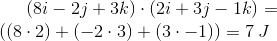# AP Physics C: Mechanics : Work

## Example Questions

### Example Question #1 : Work, Energy, And Power

A 25kg child climbs up a tree. How much work is required for him to climb up this tree to a height of three meters?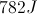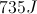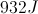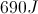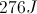Explanation:

The forces acting on the child are the force of gravity and the upward force provided by the child himself. Choosing the upward direction as positive, Newton's second law applied to the child gives the following equation.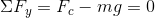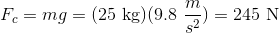To calculate the work done by the child to bring himself three meters up the tree, we use the work equation below.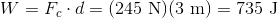### Example Question #2 : Work, Energy, And Power

A force of 27N is applied horizontally to a box resting on a level surface. The surface exerts a friction force of 2N as the box moves. If it moves a total of 3.7m, how much work was done on the box?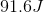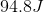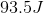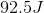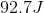Explanation:

The net force on the box is the applied force minus the friction force, since friction acts in the direction opposite motion.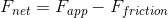Consequently, the net force is: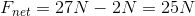The formula for work is: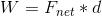Substituting in our net force and distance, we find that the work done on the box is: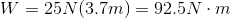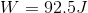### Example Question #3 : Work, Energy, And Power

An object is pushed across a rough surface with a force of 53N. The rough surface exerts a frictional force of 3.47N on the object. If the object is pushed 7.9m, how much work is done on the object?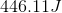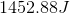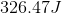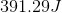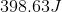Explanation:

The defintion of work is: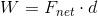The net force on this object is:We can calculate this term using the given values: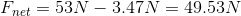The distance is given. Substituting these values: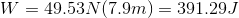### Example Question #4 : Work, Energy, And Power

ONe of the forces that act on a particle as it underjoes a dispalcement of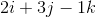m is given by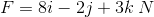.

How much work is done by that force on the particle?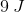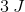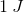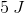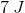Explanation:

The equation of work is given by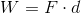, whereis force andis the distance. Both the work and the force are given to us, but in vector form. In this case, we have to take the dot product of the force and distance.

When taking dot product, keep these rule in mind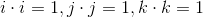Every other dot proudct is equal to 0.